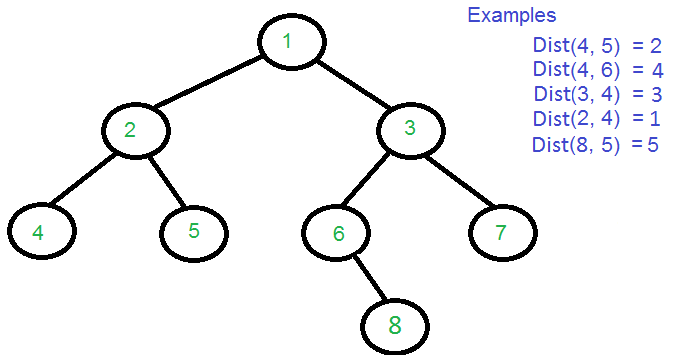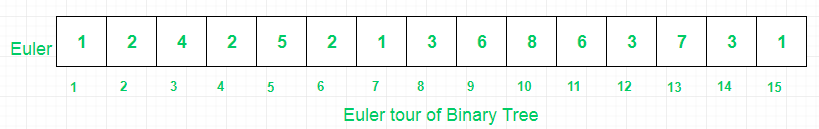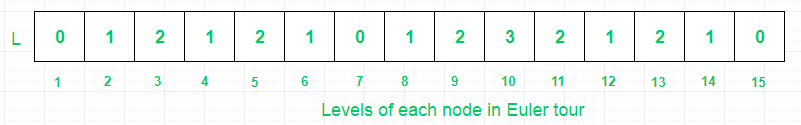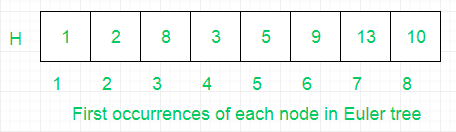# Queries to find distance between two nodes of a Binary tree

Given a binary tree, the task is to find the distance between two keys in a binary tree, no parent pointers are given. The distance between two nodes is the minimum number of edges to be traversed to reach one node from other.

We have already discussed a method which uses segment tree to reduce the query time to O(logn), here the task is to reduce query time to O(1) by compromising with space complexity to O(nlogn). In this post, we will use Sparse table instead of segment tree for finding the minimum in given range, which uses dynamic programming and bit manipulation to achieve O(1) query time.## Recommended: Please try your approach on {IDE} first, before moving on to the solution.

A sparse table will preprocess the minimum values of given range for L array in Nlogn space i.e. each node will contain chain of values of log(i) length where i is the index of the ith node in L array. Each entry in the sparse table says M[i][j] will represent the index of the minimum value in the subarray starting at i having length 2^j.

The distance between two nodes can be obtained in terms of lowest common ancestor.

```Dist(n1, n2) = Level[n1] + Level[n2] - 2*Level[lca]
```

This problem can be breakdown into:

1. Finding levels of each node
2. Finding the Euler tour of binary tree
3. Building sparse table for LCA.

These steps are explained below :

1. Find the levels of each node by applying level order traversal.
2. Find the LCA of two nodes in binary tree in O(logn) by Storing Euler tour of Binary tree in array and computing two other arrays with the help of levels of each node and Euler tour.
These steps are shown below:

(I) First, find Euler Tour of binary tree.(II) Then, store levels of each node in Euler array.(III) Then, store First occurrences of all nodes of binary tree in Euler array. H stores the indices of nodes from Euler array, so that range of query for finding minimum can be minimized and their by further optimizing the query time.3. Then build sparse table on L array and find the minimum value say X in range (H[A] to H[B]). Then, we use the index of value X as an index to Euler array to get LCA, i.e. Euler[index(X)].

Let, A=8 and B=5.
(I) H= 1 and H=2
(II) we get min value in L array between 1 and 2 as X=0, index=7
(III) Then, LCA= Euler, i.e LCA=1.

4. Finally, apply distance formula discussed above to get the distance between two nodes.

## C++

 `#include ` `#define MAX 100001 ` `using` `namespace` `std; ` ` `  `/* A tree node structure */` `struct` `Node { ` `    ``int` `data; ` `    ``struct` `Node* left; ` `    ``struct` `Node* right; ` `}; ` ` `  `/* Utility function to create a new Binary Tree node */` `struct` `Node* newNode(``int` `data) ` `{ ` `    ``struct` `Node* temp = ``new` `struct` `Node; ` `    ``temp->data = data; ` `    ``temp->left = temp->right = NULL; ` `    ``return` `temp; ` `} ` ` `  `// Array to store level of each node ` `int` `level[MAX]; ` ` `  `// Utility Function to store level of all nodes ` `void` `FindLevels(``struct` `Node* root) ` `{ ` `    ``if` `(!root) ` `        ``return``; ` ` `  `    ``// queue to hold tree node with level ` `    ``queue > q; ` ` `  `    ``// let root node be at level 0 ` `    ``q.push({ root, 0 }); ` `    ``pair<``struct` `Node*, ``int``> p; ` ` `  `    ``// Do level Order Traversal of tree ` `    ``while` `(!q.empty()) { ` `        ``p = q.front(); ` `        ``q.pop(); ` ` `  `        ``// Node p.first is on level p.second ` `        ``level[p.first->data] = p.second; ` ` `  `        ``// If left child exits, put it in queue ` `        ``// with current_level +1 ` `        ``if` `(p.first->left) ` `            ``q.push({ p.first->left, p.second + 1 }); ` ` `  `        ``// If right child exists, put it in queue ` `        ``// with current_level +1 ` `        ``if` `(p.first->right) ` `            ``q.push({ p.first->right, p.second + 1 }); ` `    ``} ` `} ` ` `  `// Stores Euler Tour ` `int` `Euler[MAX]; ` ` `  `// index in Euler array ` `int` `idx = 0; ` ` `  `// Find Euler Tour ` `void` `eulerTree(``struct` `Node* root) ` `{ ` ` `  `    ``// store current node's data ` `    ``Euler[++idx] = root->data; ` ` `  `    ``// If left node exists ` `    ``if` `(root->left) { ` ` `  `        ``// traverse left subtree ` `        ``eulerTree(root->left); ` ` `  `        ``// store parent node's data ` `        ``Euler[++idx] = root->data; ` `    ``} ` ` `  `    ``// If right node exists ` `    ``if` `(root->right) { ` ` `  `        ``// traverse right subtree ` `        ``eulerTree(root->right); ` ` `  `        ``// store parent node's data ` `        ``Euler[++idx] = root->data; ` `    ``} ` `} ` ` `  `// checks for visited nodes ` `int` `vis[MAX]; ` ` `  `// Stores level of Euler Tour ` `int` `L[MAX]; ` ` `  `// Stores indices of the first occurrence  ` `// of nodes in Euler tour ` `int` `H[MAX]; ` ` `  `// Preprocessing Euler Tour for finding LCA ` `void` `preprocessEuler(``int` `size) ` `{ ` `    ``for` `(``int` `i = 1; i <= size; i++) { ` `        ``L[i] = level[Euler[i]]; ` ` `  `        ``// If node is not visited before ` `        ``if` `(vis[Euler[i]] == 0) { ` ` `  `            ``// Add to first occurrence ` `            ``H[Euler[i]] = i; ` ` `  `            ``// Mark it visited ` `            ``vis[Euler[i]] = 1; ` `        ``} ` `    ``} ` `} ` ` `  `// Sparse table of size [MAX][LOGMAX] ` `// M[i][j] is the index of the minimum value in ` `// the sub array starting at i having length 2^j ` `int` `M[MAX]; ` ` `  `// Utility function to preprocess Sparse table ` `void` `preprocessLCA(``int` `N) ` `{ ` `    ``for` `(``int` `i = 0; i < N; i++) ` `        ``M[i] = i; ` ` `  `    ``for` `(``int` `j = 1; 1 << j <= N; j++) ` `        ``for` `(``int` `i = 0; i + (1 << j) - 1 < N; i++) ` `            ``if` `(L[M[i][j - 1]] < L[M[i + (1 << (j - 1))][j - 1]]) ` `                ``M[i][j] = M[i][j - 1]; ` `            ``else` `                ``M[i][j] = M[i + (1 << (j - 1))][j - 1]; ` `} ` ` `  `// Utility function to find the index of the minimum ` `// value in range a to b ` `int` `LCA(``int` `a, ``int` `b) ` `{ ` `    ``// Subarray of length 2^j ` `    ``int` `j = log2(b - a + 1); ` `    ``if` `(L[M[a][j]] <= L[M[b - (1 << j) + 1][j]]) ` `        ``return` `M[a][j]; ` ` `  `    ``else` `        ``return` `M[b - (1 << j) + 1][j]; ` `} ` ` `  `// Function to return distance between ` `// two nodes n1 and n2 ` `int` `findDistance(``int` `n1, ``int` `n2) ` `{ ` `    ``// Maintain original Values ` `    ``int` `prevn1 = n1, prevn2 = n2; ` ` `  `    ``// Get First Occurrence of n1 ` `    ``n1 = H[n1]; ` ` `  `    ``// Get First Occurrence of n2 ` `    ``n2 = H[n2]; ` ` `  `    ``// Swap if low>high ` `    ``if` `(n2 < n1) ` `        ``swap(n1, n2); ` ` `  `    ``// Get position of minimum value ` `    ``int` `lca = LCA(n1, n2); ` ` `  `    ``// Extract value out of Euler tour ` `    ``lca = Euler[lca]; ` ` `  `    ``// return calculated distance ` `    ``return` `level[prevn1] + level[prevn2] - 2 * level[lca]; ` `} ` ` `  `void` `preProcessing(Node* root, ``int` `N) ` `{ ` `    ``// Build Tree ` `    ``eulerTree(root); ` ` `  `    ``// Store Levels ` `    ``FindLevels(root); ` ` `  `    ``// Find L and H array ` `    ``preprocessEuler(2 * N - 1); ` ` `  `    ``// Build sparse table ` `    ``preprocessLCA(2 * N - 1); ` `} ` ` `  `/* Driver function to test above functions */` `int` `main() ` `{ ` `    ``// Number of nodes ` `    ``int` `N = 8; ` ` `  `    ``/* Constructing tree given in the above figure */` `    ``Node* root = newNode(1); ` `    ``root->left = newNode(2); ` `    ``root->right = newNode(3); ` `    ``root->left->left = newNode(4); ` `    ``root->left->right = newNode(5); ` `    ``root->right->left = newNode(6); ` `    ``root->right->right = newNode(7); ` `    ``root->right->left->right = newNode(8); ` ` `  `    ``// Function to do all preprocessing ` `    ``preProcessing(root, N); ` ` `  `    ``cout << ``"Dist(4, 5) = "` `<< findDistance(4, 5) << ``"\n"``; ` `    ``cout << ``"Dist(4, 6) = "` `<< findDistance(4, 6) << ``"\n"``; ` `    ``cout << ``"Dist(3, 4) = "` `<< findDistance(3, 4) << ``"\n"``; ` `    ``cout << ``"Dist(2, 4) = "` `<< findDistance(2, 4) << ``"\n"``; ` `    ``cout << ``"Dist(8, 5) = "` `<< findDistance(8, 5) << ``"\n"``; ` ` `  `    ``return` `0; ` `} `

## Java

 `// Java implementation of the approach ` `import` `java.util.*; ` ` `  `class` `GFG { ` ` `  `    ``static` `class` `Pair { ` `        ``T first; ` `        ``V second; ` ` `  `        ``Pair() { ` `        ``} ` ` `  `        ``Pair(T first, V second) { ` `            ``this``.first = first; ` `            ``this``.second = second; ` `        ``} ` `    ``} ` ` `  `    ``static` `class` `Node { ` `        ``int` `data; ` `        ``Node left, right; ` ` `  `        ``Node(``int` `data) { ` `            ``this``.data = data; ` `            ``this``.left = ``this``.right = ``null``; ` `        ``} ` `    ``} ` ` `  `    ``static` `int` `MAX = ``100001``; ` ` `  `    ``// Array to store level of each node ` `    ``static` `int``[] level = ``new` `int``[MAX]; ` ` `  `    ``// Utility Function to store level of all nodes ` `    ``static` `void` `FindLevels(Node root) { ` `        ``if` `(root == ``null``) ` `            ``return``; ` ` `  `        ``// queue to hold tree node with level ` `        ``Queue> q = ``new` `LinkedList<>(); ` ` `  `        ``// let root node be at level 0 ` `        ``q.add(``new` `Pair<>(root, ``0``)); ` `        ``Pair p = ``new` `Pair<>(); ` ` `  `        ``// Do level Order Traversal of tree ` `        ``while` `(!q.isEmpty()) { ` `            ``p = q.poll(); ` ` `  `            ``// Node p.first is on level p.second ` `            ``level[p.first.data] = p.second; ` ` `  `            ``// If left child exits, put it in queue ` `            ``// with current_level +1 ` `            ``if` `(p.first.left != ``null``) ` `                ``q.add(``new` `Pair<>(p.first.left, p.second + ``1``)); ` ` `  `            ``// If right child exists, put it in queue ` `            ``// with current_level +1 ` `            ``if` `(p.first.right != ``null``) ` `                ``q.add(``new` `Pair<>(p.first.right, p.second + ``1``)); ` `        ``} ` `    ``} ` ` `  `    ``// Stores Euler Tour ` `    ``static` `int``[] Euler = ``new` `int``[MAX]; ` ` `  `    ``// index in Euler array ` `    ``static` `int` `idx = ``0``; ` ` `  `    ``// Find Euler Tour ` `    ``static` `void` `eulerTree(Node root) { ` ` `  `        ``// store current node's data ` `        ``Euler[++idx] = root.data; ` ` `  `        ``// If left node exists ` `        ``if` `(root.left != ``null``) { ` ` `  `            ``// traverse left subtree ` `            ``eulerTree(root.left); ` ` `  `            ``// store parent node's data ` `            ``Euler[++idx] = root.data; ` `        ``} ` ` `  `        ``// If right node exists ` `        ``if` `(root.right != ``null``) { ` ` `  `            ``// traverse right subtree ` `            ``eulerTree(root.right); ` ` `  `            ``// store parent node's data ` `            ``Euler[++idx] = root.data; ` `        ``} ` `    ``} ` ` `  `    ``// checks for visited nodes ` `    ``static` `int``[] vis = ``new` `int``[MAX]; ` ` `  `    ``// Stores level of Euler Tour ` `    ``static` `int``[] L = ``new` `int``[MAX]; ` ` `  `    ``// Stores indices of the first occurrence ` `    ``// of nodes in Euler tour ` `    ``static` `int``[] H = ``new` `int``[MAX]; ` ` `  `    ``// Preprocessing Euler Tour for finding LCA ` `    ``static` `void` `preprocessEuler(``int` `size) { ` `        ``for` `(``int` `i = ``1``; i <= size; i++) { ` `            ``L[i] = level[Euler[i]]; ` ` `  `            ``// If node is not visited before ` `            ``if` `(vis[Euler[i]] == ``0``) { ` ` `  `                ``// Add to first occurrence ` `                ``H[Euler[i]] = i; ` ` `  `                ``// Mark it visited ` `                ``vis[Euler[i]] = ``1``; ` `            ``} ` `        ``} ` `    ``} ` ` `  `    ``// Sparse table of size [MAX][LOGMAX] ` `    ``// M[i][j] is the index of the minimum value in ` `    ``// the sub array starting at i having length 2^j ` `    ``static` `int``[][] M = ``new` `int``[MAX][``18``]; ` ` `  `    ``// Utility function to preprocess Sparse table ` `    ``static` `void` `preprocessLCA(``int` `N) { ` `        ``for` `(``int` `i = ``0``; i < N; i++) ` `            ``M[i][``0``] = i; ` ` `  `        ``for` `(``int` `j = ``1``; ``1` `<< j <= N; j++) ` `            ``for` `(``int` `i = ``0``; i + (``1` `<< j) - ``1` `< N; i++) ` `                ``if` `(L[M[i][j - ``1``]] < L[M[i + (``1` `<< (j - ``1``))][j - ``1``]]) ` `                    ``M[i][j] = M[i][j - ``1``]; ` `                ``else` `                    ``M[i][j] = M[i + (``1` `<< (j - ``1``))][j - ``1``]; ` `    ``} ` ` `  `    ``// Utility function to find the index of the minimum ` `    ``// value in range a to b ` `    ``static` `int` `LCA(``int` `a, ``int` `b) { ` `        ``// Subarray of length 2^j ` `        ``int` `j = (``int``) (Math.log(b - a + ``1``) / Math.log(``2``)); ` `        ``if` `(L[M[a][j]] <= L[M[b - (``1` `<< j) + ``1``][j]]) ` `            ``return` `M[a][j]; ` ` `  `        ``else` `            ``return` `M[b - (``1` `<< j) + ``1``][j]; ` `    ``} ` ` `  `    ``// Function to return distance between ` `    ``// two nodes n1 and n2 ` `    ``static` `int` `findDistance(``int` `n1, ``int` `n2) { ` `        ``// Maintain original Values ` `        ``int` `prevn1 = n1, prevn2 = n2; ` ` `  `        ``// Get First Occurrence of n1 ` `        ``n1 = H[n1]; ` ` `  `        ``// Get First Occurrence of n2 ` `        ``n2 = H[n2]; ` ` `  `        ``// Swap if low>high ` `        ``if` `(n2 < n1) { ` `            ``int` `temp = n1; ` `            ``n1 = n2; ` `            ``n2 = temp; ` `        ``} ` ` `  `        ``// Get position of minimum value ` `        ``int` `lca = LCA(n1, n2); ` ` `  `        ``// Extract value out of Euler tour ` `        ``lca = Euler[lca]; ` ` `  `        ``// return calculated distance ` `        ``return` `level[prevn1] + level[prevn2] - ``2` `* level[lca]; ` `    ``} ` ` `  `    ``static` `void` `preProcessing(Node root, ``int` `N) { ` `        ``// Build Tree ` `        ``eulerTree(root); ` ` `  `        ``// Store Levels ` `        ``FindLevels(root); ` ` `  `        ``// Find L and H array ` `        ``preprocessEuler(``2` `* N - ``1``); ` ` `  `        ``// Build sparse table ` `        ``preprocessLCA(``2` `* N - ``1``); ` `    ``} ` ` `  `    ``// Driver Code ` `    ``public` `static` `void` `main(String[] args) { ` `        ``// Number of nodes ` `        ``int` `N = ``8``; ` ` `  `        ``/* Constructing tree given in the above figure */` `        ``Node root = ``new` `Node(``1``); ` `        ``root.left = ``new` `Node(``2``); ` `        ``root.right = ``new` `Node(``3``); ` `        ``root.left.left = ``new` `Node(``4``); ` `        ``root.left.right = ``new` `Node(``5``); ` `        ``root.right.left = ``new` `Node(``6``); ` `        ``root.right.right = ``new` `Node(``7``); ` `        ``root.right.left.right = ``new` `Node(``8``); ` ` `  `        ``// Function to do all preprocessing ` `        ``preProcessing(root, N); ` ` `  `        ``System.out.println(``"Dist(4, 5) = "` `+ findDistance(``4``, ``5``)); ` `        ``System.out.println(``"Dist(4, 6) = "` `+ findDistance(``4``, ``6``)); ` `        ``System.out.println(``"Dist(3, 4) = "` `+ findDistance(``3``, ``4``)); ` `        ``System.out.println(``"Dist(2, 4) = "` `+ findDistance(``2``, ``4``)); ` `        ``System.out.println(``"Dist(8, 5) = "` `+ findDistance(``8``, ``5``)); ` `    ``} ` `} ` ` `  `// This code is contributed by ` `// sanjeev2552 `

## Python3

 `from` `collections ``import` `deque ` `from` `math ``import` `log2 ` ` `  `MAX` `=` `100001` ` `  `# A tree node structure ` `class` `Node: ` `    ``def` `__init__(``self``, data): ` `        ``self``.data ``=` `data ` `        ``self``.left ``=` `None` `        ``self``.right ``=` `None` ` `  `# Array to store level of each node ` `level ``=` `[``0``] ``*` `MAX` ` `  `# Utility Function to store level of all nodes ` `def` `findLevels(root: Node): ` `    ``global` `level ` ` `  `    ``if` `root ``is` `None``: ` `        ``return` ` `  `    ``# queue to hold tree node with level ` `    ``q ``=` `deque() ` ` `  `    ``# let root node be at level 0 ` `    ``q.append((root, ``0``)) ` ` `  `    ``# Do level Order Traversal of tree ` `    ``while` `q: ` `        ``p ``=` `q[``0``] ` `        ``q.popleft() ` ` `  `        ``# Node p.first is on level p.second ` `        ``level[p[``0``].data] ``=` `p[``1``] ` ` `  `        ``# If left child exits, put it in queue ` `        ``# with current_level +1 ` `        ``if` `p[``0``].left: ` `            ``q.append((p[``0``].left, p[``1``] ``+` `1``)) ` ` `  `        ``# If right child exists, put it in queue ` `        ``# with current_level +1 ` `        ``if` `p[``0``].right: ` `            ``q.append((p[``0``].right, p[``1``] ``+` `1``)) ` ` `  `# Stores Euler Tour ` `Euler ``=` `[``0``] ``*` `MAX` ` `  `# index in Euler array ` `idx ``=` `0` ` `  `# Find Euler Tour ` `def` `eulerTree(root: Node): ` `    ``global` `Euler, idx ` `    ``idx ``+``=` `1` ` `  `    ``# store current node's data ` `    ``Euler[idx] ``=` `root.data ` ` `  `    ``# If left node exists ` `    ``if` `root.left: ` ` `  `        ``# traverse left subtree ` `        ``eulerTree(root.left) ` `        ``idx ``+``=` `1` ` `  `        ``# store parent node's data ` `        ``Euler[idx] ``=` `root.data ` ` `  `    ``# If right node exists ` `    ``if` `root.right: ` ` `  `        ``# traverse right subtree ` `        ``eulerTree(root.right) ` `        ``idx ``+``=` `1` ` `  `        ``# store parent node's data ` `        ``Euler[idx] ``=` `root.data ` ` `  `# checks for visited nodes ` `vis ``=` `[``0``] ``*` `MAX` ` `  `# Stores level of Euler Tour ` `L ``=` `[``0``] ``*` `MAX` ` `  `# Stores indices of the first occurrence ` `# of nodes in Euler tour ` `H ``=` `[``0``] ``*` `MAX` ` `  `# Preprocessing Euler Tour for finding LCA ` `def` `preprocessEuler(size: ``int``): ` `    ``global` `L, H, vis ` `    ``for` `i ``in` `range``(``1``, size ``+` `1``): ` `        ``L[i] ``=` `level[Euler[i]] ` ` `  `        ``# If node is not visited before ` `        ``if` `vis[Euler[i]] ``=``=` `0``: ` ` `  `            ``# Add to first occurrence ` `            ``H[Euler[i]] ``=` `i ` ` `  `            ``# Mark it visited ` `            ``vis[Euler[i]] ``=` `1` ` `  `# Sparse table of size [MAX][LOGMAX] ` `# M[i][j] is the index of the minimum value in ` `# the sub array starting at i having length 2^j ` `M ``=` `[[``0` `for` `i ``in` `range``(``18``)] ``for` `j ``in` `range``(``MAX``)] ` ` `  `# Utility function to preprocess Sparse table ` `def` `preprocessLCA(N: ``int``): ` `    ``global` `M ` `    ``for` `i ``in` `range``(N): ` `        ``M[i][``0``] ``=` `i ` ` `  `    ``j ``=` `1` `    ``while` `1` `<< j <``=` `N: ` `        ``i ``=` `0` `        ``while` `i ``+` `(``1` `<< j) ``-` `1` `< N: ` `            ``if` `L[M[i][j ``-` `1``]] < L[M[i ``+`  `                ``(``1` `<< (j ``-` `1``))][j ``-` `1``]]: ` `                ``M[i][j] ``=` `M[i][j ``-` `1``] ` `            ``else``: ` `                ``M[i][j] ``=` `M[i ``+` `(``1` `<< (j ``-` `1``))][j ``-` `1``] ` `            ``i ``+``=` `1` `        ``j ``+``=` `1` ` `  `# Utility function to find the index of the minimum ` `# value in range a to b ` `def` `LCA(a: ``int``, b: ``int``) ``-``> ``int``: ` ` `  `    ``# Subarray of length 2^j ` `    ``j ``=` `int``(log2(b ``-` `a ``+` `1``)) ` `    ``if` `L[M[a][j]] <``=` `L[M[b ``-` `(``1` `<< j) ``+` `1``][j]]: ` `        ``return` `M[a][j] ` `    ``else``: ` `        ``return` `M[b ``-` `(``1` `<< j) ``+` `1``][j] ` ` `  `# Function to return distance between ` `# two nodes n1 and n2 ` `def` `findDistance(n1: ``int``, n2: ``int``) ``-``> ``int``: ` ` `  `    ``# Maintain original Values ` `    ``prevn1 ``=` `n1 ` `    ``prevn2 ``=` `n2 ` ` `  `    ``# Get First Occurrence of n1 ` `    ``n1 ``=` `H[n1] ` ` `  `    ``# Get First Occurrence of n2 ` `    ``n2 ``=` `H[n2] ` ` `  `    ``# Swap if low>high ` `    ``if` `n2 < n1: ` `        ``n1, n2 ``=` `n2, n1 ` ` `  `    ``# Get position of minimum value ` `    ``lca ``=` `LCA(n1, n2) ` ` `  `    ``# Extract value out of Euler tour ` `    ``lca ``=` `Euler[lca] ` ` `  `    ``# return calculated distance ` `    ``return` `level[prevn1] ``+` `level[prevn2] ``-` `2` `*` `level[lca] ` ` `  `def` `preProcessing(root: Node, N: ``int``): ` ` `  `    ``# Build Tree ` `    ``eulerTree(root) ` ` `  `    ``# Store Levels ` `    ``findLevels(root) ` ` `  `    ``# Find L and H array ` `    ``preprocessEuler(``2` `*` `N ``-` `1``) ` ` `  `    ``# Build sparse table ` `    ``preprocessLCA(``2` `*` `N ``-` `1``) ` ` `  `# Driver Code ` `if` `__name__ ``=``=` `"__main__"``: ` ` `  `    ``# Number of nodes ` `    ``N ``=` `8` ` `  `    ``# Constructing tree given in the above figure ` `    ``root ``=` `Node(``1``) ` `    ``root.left ``=` `Node(``2``) ` `    ``root.right ``=` `Node(``3``) ` `    ``root.left.left ``=` `Node(``4``) ` `    ``root.left.right ``=` `Node(``5``) ` `    ``root.right.left ``=` `Node(``6``) ` `    ``root.right.right ``=` `Node(``7``) ` `    ``root.right.left.right ``=` `Node(``8``) ` ` `  `    ``# Function to do all preprocessing ` `    ``preProcessing(root, N) ` ` `  `    ``print``(``"Dist(4, 5) ="``, findDistance(``4``, ``5``)) ` `    ``print``(``"Dist(4, 6) ="``, findDistance(``4``, ``6``)) ` `    ``print``(``"Dist(3, 4) ="``, findDistance(``3``, ``4``)) ` `    ``print``(``"Dist(2, 4) ="``, findDistance(``2``, ``4``)) ` `    ``print``(``"Dist(8, 5) ="``, findDistance(``8``, ``5``)) ` ` `  `# This code is contributed by ` `# sanjeev2552 `

Output:

```Dist(4, 5) = 2
Dist(4, 6) = 4
Dist(3, 4) = 3
Dist(2, 4) = 1
Dist(8, 5) = 5
```

Time Complexity: O(1)
Space Complexity: O(N log N)My Personal Notes arrow_drop_upCheck out this Author's contributed articles.

If you like GeeksforGeeks and would like to contribute, you can also write an article using contribute.geeksforgeeks.org or mail your article to contribute@geeksforgeeks.org. See your article appearing on the GeeksforGeeks main page and help other Geeks.

Please Improve this article if you find anything incorrect by clicking on the "Improve Article" button below.

1

Please write to us at contribute@geeksforgeeks.org to report any issue with the above content.